# Non Verbal Reasoning - Cubes and Dice

Exercise : Cubes and Dice - Section 1
Directions to Solve

The sheet of paper shown in the figure (X) given on the left hand side, in each problem, is folded to form a box. Choose from amongst the alternatives (1), (2), (3) and (4), the boxes that are similar to the box that will be formed.

1.

Choose the box that is similar to the box formed from the given sheet of paper (X).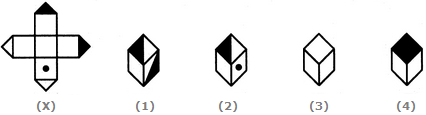1 and 2 only
2 and 4 only
2 and 3 only
1 and 4 only
Explanation:
The fig. (X) is similar to the Form VI. So, when a cube is formed by folding the sheet shown in fig. (X), then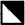is one of the faces of the cube. However, the cube in fig. (1) has two such faces and fig. (4) has a face which is completely shaded. So, these two cubes cannot be formed. Hence, only the cubes in figures (2) and (3) can be formed.

2.

Choose the box that is similar to the box formed from the given sheet of paper (X).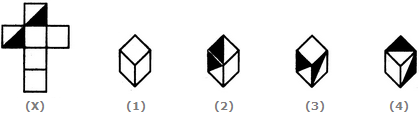1 and 4 only
3 and 4 only
1 and 2 only
2 and 3 only
Explanation:
The fig. (X) is similar to the Form I. So, when the sheet shown in fig. (X) is folded to form a cube then one of the two half-shaded faces lies opposite to one of the blank faces and the other half-shaded face lies opposite to another blank face. The two remaining blank faces lie opposite to each other. Thus, both the cubes shown in figures (1).and (4) can be formed when the sheet shown in fig. (X) is folded. Also, though the cubes shown in figures (2) and (3) have faces that can appear adjacent to each other but the cube formed by folding the sheet in fig. (X) cannot be rotated to form either of the two. Hence, the cubes in figures (2) and (3) cannot be formed.

3.

How many dots lie opposite to the face having three dots, when the given figure is folded to form a cube?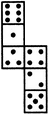2
4
5
6
Explanation:
The given figure is similar to Form V. Therefore, when this figure is folded to form a cube then the face bearing six dots will lie opposite the face bearing three dots.

4.

Choose the box that is similar to the box formed from the given sheet of paper (X).1 and 3 only
1 and 4 only
2 and 4 only
3 and 4 only
Explanation:
The fig. (X) is similar to Form II. So, when the sheet shown in fig. (X) is folded to form a cube then the two half-shaded faces lie opposite to each other, the face bearing a circle lies opposite to one of the two blank faces and the two remaining blank faces lie opposite to each other. Therefore, the cubes shown in fig. (4) which has the two half-shaded faces adjacent to each other, cannot be formed by folding the sheet shown in fig. (X). Also, the cube shown in fig. (2) has the face bearing a circle adjacent to two blank faces. This is not possible since there is one blank face opposite to the circle and one blank face opposite to the third blank face. Hence, only the cubes in figures (1) and (3) can be formed.

5.

Choose the box that is similar to the box formed from the given sheet of paper (X).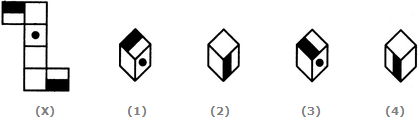1 and 2 only
2 and 3 only
2 and 4 only
1, 2, 3 and 4